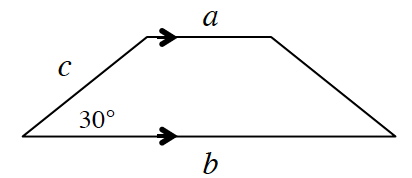### Home > CCG > Chapter 10 > Lesson 10.2.2 > Problem10-91

10-91.

Multiple Choice: Which expression represents the area of the trapezoid right?1. $\frac{c\left(a+b\right)}{4}$

1. $\frac{c\left(a+b\right)}{2}$

1. $\frac { b c } { 2 }$

1. $\frac { a + b + c } { 2 }$

1. None of these

(a)